Introduction to Statistical Modeling with SAS/STAT Software

### Regression Models and Models with Classification Effects

A linear regression model in the broad sense has the form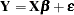where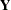is the vector of response values,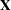is the matrix of regressor effects,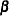is the vector of regression parameters, andis the vector of errors or residuals. A regression model in the narrow sense—as compared to a classification model—is a linear model in which all regressor effects are continuous variables. In other words, each effect in the model contributes a single column to thematrix and a single parameter to the overall model. For example, a regression of subjects’ weight (
) on the regressors age (
) and body mass index (bmi,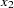) is a regression model in this narrow sense. In symbolic notation you can write this regression model as

weight = age + bmi + error

This symbolic notation expands into the statistical model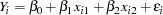Single parameters are used to model the effects of age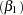and bmi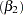, respectively.

A classification effect, on the other hand, is associated with possibly more than one column of thematrix. Classification with respect to a variable is the process by which each observation is associated with one oflevels; the process of determining theselevels is referred to as levelization of the variable. Classification variables are used in models to identify experimental conditions, group membership, treatments, and so on. The actual values of the classification variable are not important, and the variable can be a numeric or a character variable. What is important is the association of discrete values or levels of the classification variable with groups of observations. For example, in the previous illustration, if the regression also takes into account the subjects’ gender, this can be incorporated in the model with a two-level classification variable. Suppose that the values of the gender variable are coded as "F" and "M," respectively. In symbolic notation the model

weight = age + bmi + gender + error

expands into the statistical model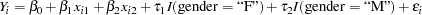where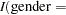"F"is the indicator function that returnsif the value of the gender variable is "F" andotherwise. Parameters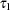and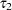are associated with the gender classification effect. This form of parameterizing the gender effect in the model is only one of several different methods of incorporating the levels of a classification variable in the model. This form, the so-called singular parameterization, is the most general approach, and it is used in the GLM, MIXED, and GLIMMIX procedures. Alternatively, classification effects with various forms of nonsingular parameterizations are available in such procedures as GENMOD and LOGISTIC. See the documentation for the individual SAS/STAT procedures on their respective facilities for parameterizing classification variables and the section Parameterization of Model Effects in Chapter 18, Shared Concepts and Topics, for general details.

Models that contain only classification effects are often identified with analysis of variance (ANOVA) models, because ANOVA methods are frequently used in their analysis. This is particularly true for experimental data where the model effects comprise effects of the treatment and error-control design. However, classification effects appear more widely than in models to which analysis of variance methods are applied. For example, many mixed models, where parameters are estimated by restricted maximum likelihood, consist entirely of classification effects but do not permit the sum of squares decomposition typical for ANOVA techniques.

Many models contain both continuous and classification effects. For example, a continuous-by-class effect consists of at least one continuous variable and at least one classification variable. Such effects are convenient, for example, to vary slopes in a regression model by the levels of a classification variable. Also, recent enhancements to linear modeling syntax in some SAS/STAT procedures (including GLIMMIX and GLMSELECT) enable you to construct sets of columns inmatrices from a single continuous variable. An example is modeling with splines where the values of a continuous variable x are expanded into a spline basis that occupies multiple columns in thematrix. For purposes of the analysis you can treat these columns as a single unit or as individual, unrelated columns. For more details, see the section Constructed Effects and the EFFECT Statement in Chapter 18, Shared Concepts and Topics.Previous Page | Next Page | Top of Page